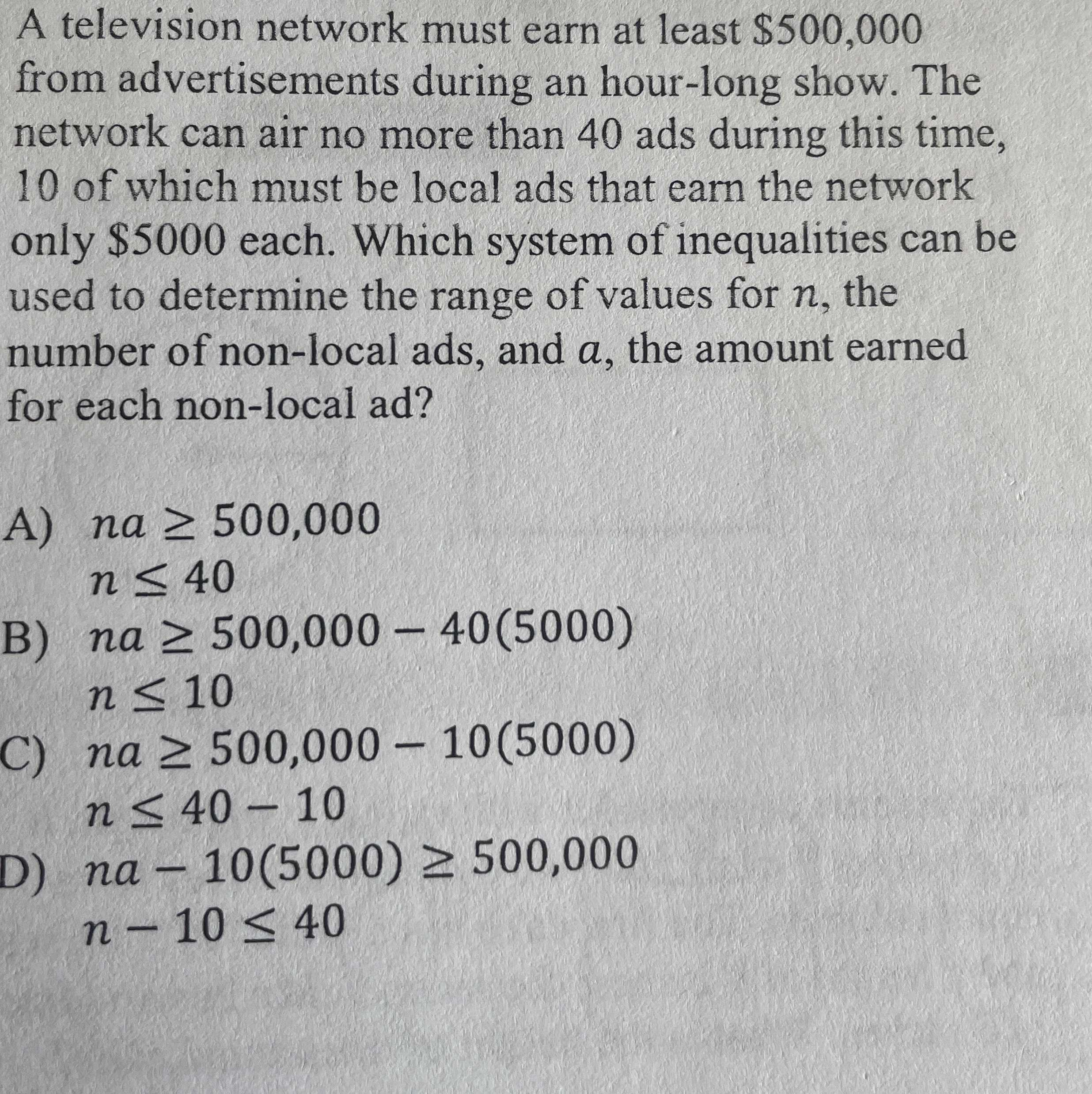### Still have math questions?

Algebra
QuestionA television network must earn at least $$\ 500,000$$ from advertisements during an hour-long show. The network can air no more than $$40$$ ads during this time, $$10$$ of which must be local ads that earn the network only $$\ 5000$$ each. Which system of inequalities can be used to determine the range of values for $$n$$ , the number of non-local ads, and a, the amount earned for each non-local ad?

A) $$n a \geq 500,000$$

$$n \leq 40$$

B) $$n a \geq 500,000 - 40 ( 5000 )$$

$$n \leq 10$$

C) $$n a \geq 00,000 - 10 ( 5000 )$$

$$n \leq 40 - 10$$

D) $$n a - 10 ( 5000 ) \geq 500,000$$

$$n - 10 \leq 40$$